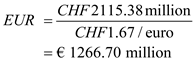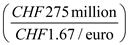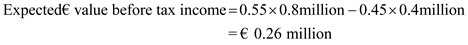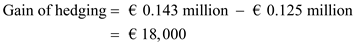# Quiz 17: Risk Management and the Foreign Currency Hedging Decision

Business

Given information: The company CR has a Swiss project. The earnings from the project is CHF250 million or CHF300 million per year indefinitely. Company CR has discounting rate of 13%. And the cost of project is euro 1,100 million. The current exchange rate is CHF1.67per euro. Calculate the expected value of cash flow each year by taking the average of probable earnings per year as follows: Per year probable earningsAs the discount rate is 13% p.a., present value of project will be, the probable earnings divided by rate of discounting (Summation of Geometric Progression of Infinite terms):Since the current exchange rate is CHF 1.67/EUR; hence present value of project in terms of will be:Cost of the project is € 1100 million. Hence, Net Present Value of the project is € 166.70 million (€ 1266.70 - € 1100). As NPV of the project is positive and hence, it should accept the project No, it will not have any problem in hedging the CHF cash flows because annual line of credit required to hedge CHF 275 million is €164.67 million, While the available line of credit is of €400 million.

The main objective of hedging is to reduce uncertainty of a firm's future cash flow. Owner of a business being a risk-averse; always want to avoid any deviation in the expected profit of a business in a year. For example: Mr. A is having a business which is having currency risk (trade with foreign nations). Mr. A will always try to forecast a profit for the year at the beginning of the year. Now, his forecast can be meet only if there is no future fluctuation in the currency rate as forecasted by him (which is very rare !) and hence, any deviation in exchange rate can decrease his profit and hence, can deviate his future plans. To avoid these, he will prefer to hedge.

Given information: The project will earn £20 million of revenue in one year. The project's cost is €30million and it will be paid in one year. The spot rate in year-1 will be €1.54/£ with 55% probability or €1.48/£ with 45% probability. French tax rate is 45 percent on positive income and firm's losses are refundable at 35% rate. a. Calculate unhedged project's after-tax income by referring below steps: Calculate £by as follows:If the cash flow is un-hedged, company will experience following income on project: Return from project with 55% certainty:Return from project with 45% certainty:Hence, expected € value before tax income on un-hedged project will be:The expected value of after tax income on un-hedged project will be:. Thus, the expected value of after tax income on un-hedged project will be €0.125millio. b. If company fully hedged the exchange rate risk, it will receive:Hence, the company will have an income of € 0.26 million before tax (difference between earnings and cost). Hence, after tax income will be following:Thus, the after tax income of the hedged project is €0.143million. c. Calculate gain of hedging. Gain on hedging over un-hedged position will be the differences between after tax income of hedge position and after tax income of unhedge position: Calculate as below:Thus, the gain of hedging is €18,000.

There is no answer for this question

There is no answer for this question

There is no answer for this question